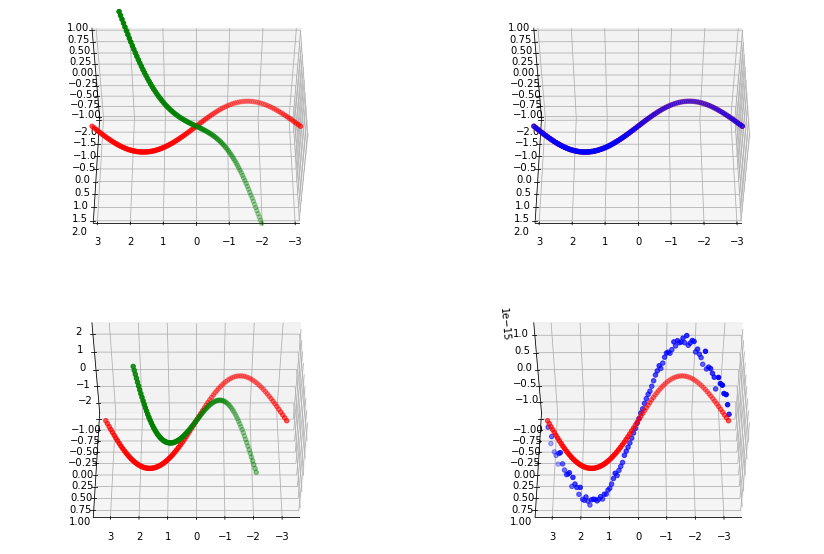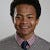# Regressing sinusoids for a given number of iterations

On a previous series of posts I wrote about how using a small batch size led to better results on the task of regressing a sinusoid using stochastic gradient descent. https://fulkast.medium.com/sinusoid-regression-the-stats-receipts-87785297eb7c

However, I wasn't fully convinced that the smaller batch size was the sole cause of the better performance. Because…

# The Kabsch AlgorithmTop left we show the original points in red and the rotated points in green. On the top right we see the original points in red and the rectified points in blue. Note that due to the similarity, this plot appears to only show one line. On the images in the bottom row, we show the same information as in the first row, but allow the plotting library to determine the appropriate plotting scale.

In this post, I finally cover the Kabsch algorithm which can be used to retrieve the rigid body transform between two sets of points (with known 1-to-1 correspondences).

The algorithm is pretty straightforward and I've actually just followed the wikipedia description almost word for word: https://en.wikipedia.org/wiki/Kabsch_algorithm

In the toy example…

# From Graham-Schmidt To SO(n)

Previously, I wanted to write about a rotation calculation technique but realised that I should first write about generating random rotation matrices, hence my post: Graham Schmidt Orthogonalisation

Actually, that post didn't quite cover generating rotation matrices since the Graham Schmidt Orthogonalisation can only generate orthonormal/orthogonal matrices but doesn't guarantee…

# Graham Schmidt Orthogonalisation

I was gonna start out tonight writing about the Kabsch algorithm for optimal rotation calculation between two sets of points. But then I thought it would be nice to have a random rotation matrix generation procedure, so I can properly test the Kabsch algorithm I implement. …

# Sinusoid Regression: The Stats (Receipts)

On my previous post, I covered how performing gradient descent on a small batch size (e.g. 2 samples at a time) yielded better results that running over the entire dataset. Well, there are still a few "knobs" to account for before I can say that I've made a fair comparison…

# Sinusoid Regression Using Stochastic Gradient Descent (For real this time)

On a previous post (https://fulkast.medium.com/a-simple-exercise-in-regressing-a-sinusoidal-function-9e2932031155) I wrote about regressing a sinusoid using some appropriately sampled data (within the required Nyqvist frequency requirement). For the optimisation framework I chose PyTorch (for convenience) and for the optimiser I picked the "SGD" algorithm. This however, was a lie — I was not using…

# A simple exercise in regressing a sinusoidal function

My goal is simple: To learn the frequency of a sinusoid using stochastic gradient descent (SGD) in PyTorch. Periodic signals are abundant in nature, e.g. in sound signals and it would be interesting to see what simple models we can build to generate such signals.

PyTorch feels like an easy…

Rasterising a Simple Circular Signed Distance Function

In this post I am going to play with a simple example of implicit surface representation, using the signed distance function.

The signed distance function could be an efficient way of storing shape primitives. It is also composable, with other signed distance functions, to create more exciting shapes.

In the example above, I generate a simple 2-D circle and visualise its signed distance function in both 2-D and 3-D. The second 2-D image shows a binary separation between regions that are strictly positive and those that aren't. The interior of the circle has negative values.

# The trivial linear least squares problem

In this instance we are interested in solving a trivial problem with a very powerful tool. We have a target value for single variable x. …